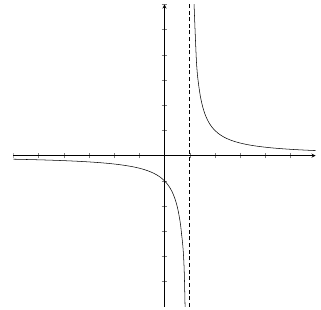# Rational Function: Definition, Equation & Examples

An error occurred trying to load this video.

Try refreshing the page, or contact customer support.

Coming up next: Perimeter of Triangles and Rectangles

### You're on a roll. Keep up the good work!

Replay
Your next lesson will play in 10 seconds
• 0:01 Definition
• 0:53 Examples of Rational Functions
• 2:02 Non-Examples of…
• 2:39 Vertical…
• 3:44 Examples
• 5:54 Lesson Summary

Want to watch this again later?

Timeline
Autoplay
Autoplay
Speed

#### Recommended Lessons and Courses for You

Lesson Transcript
Instructor: Peter Kosek

Peter has taught Mathematics at the college level and has a master's degree in Mathematics.

Rational functions are an extremely useful type of function found in mathematics. They are functions that are fractions whose numerator and denominator are both polynomials. Learn all about them in this lesson!

## Definition of a Rational Function

A rational function is a function that is a fraction and has the property that both its numerator and denominator are polynomials. In other words, R(x) is a rational function if R(x) = p(x) / q(x) where p(x) and q(x) are both polynomials. Recall that a polynomial is any function of the form f(x) = a-sub-zero + a-sub-1 times x + a-sub-2 times x^2 + . . . + a-sub-n * x^n, where a-sub-0, a-sub-1, . . ., a-sub-n are all real numbers and the exponents of each x is a non-negative integer.

## Examples of Rational Functions

The definition you just got might be a little overbearing, so let's look at some examples of rational functions:

The function R(x) = (x^2 + 4x - 1) / (3x^2 - 9x + 2) is a rational function since the numerator, x^2 + 4x - 1, is a polynomial and the denominator, 3x^2 - 9x + 2 is also a polynomial.

The function R(x) = (-2x^5 + 4x^2 - 1) / x^9 is a rational function since the numerator, -2x^5 + 4x^2 - 1, is a polynomial and the denominator, x^9, is also a polynomial.

The function R(x) = 1 / ((x - 1)(x^2 + 3)) is a rational function since the numerator, 1, is a polynomial (yes, a constant is still a polynomial) and the denominator, (x - 1)(x^2 + 3), is also a polynomial (it's just in a factored form).

## Non-Examples of Rational Functions

The function R(x) = (sqrt(x) + x^2) / (3x^2 - 9x + 2) is not a rational function since the numerator, sqrt(x) + x^2, is not a polynomial since the exponent of x is not an integer.

The function R(x) = (x - 4) / x^(-2/3) + 4 is not a rational function since the denominator, x^(-2/3) + 4, is not a polynomial since the exponent of x is not a non-negative integer.

## Vertical Asymptotes

One of the most unique properties of a rational function is that it may have vertical asymptotes. First off, we should probably define a vertical asymptote. A vertical asymptote at a value x is when the value of our function approaches either positive or negative infinity when we evaluate our function at values that approach x (but are not equal to x).

This example may help clarify the idea of a vertical asymptote:We see there is a vertical asymptote when x = 1 since the function is approaching negative infinity as we approach 1 from the left, and the function is approaching positive infinity as we approach 1 from the right.

## Finding Vertical Asymptotes

How do we find the vertical asymptotes (if any exist) if we have been given a rational function? We can use the following theorem:

Theorem: Let R(x) be a rational function with no common factors between the numerator and the denominator. Then, the real values of x that make our denominator equal to 0 will have vertical asymptotes.

## Examples of Finding Them

Let's use this theorem to find vertical asymptotes!

Find all vertical asymptotes of the function:

R(x) = (-2x^3 + 4x^2 - 1) / (x^2 + x)

To unlock this lesson you must be a Study.com Member.

### Register to view this lesson

Are you a student or a teacher?

### Unlock Your Education

#### See for yourself why 30 million people use Study.com

##### Become a Study.com member and start learning now.
Back
What teachers are saying about Study.com

### Earning College Credit

Did you know… We have over 160 college courses that prepare you to earn credit by exam that is accepted by over 1,500 colleges and universities. You can test out of the first two years of college and save thousands off your degree. Anyone can earn credit-by-exam regardless of age or education level.